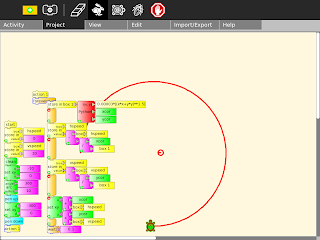## Tuesday, June 14, 2011

### Orbital motionLaw of gravity

f=G ma
mb/r2
a=G ma/r2
ay=a sin(theta)
ay=a y/r
ay=G ma y/r3
r=(x2+y2)0.5
ax=Gmax/(x2+y2)1.5

ay=Gmay/(x2+y2)1.5

Then for each time step
Vx=Vx+ax
Vy=Vy+ay
x=x+Vx
y=y+V

Labels: , , ,